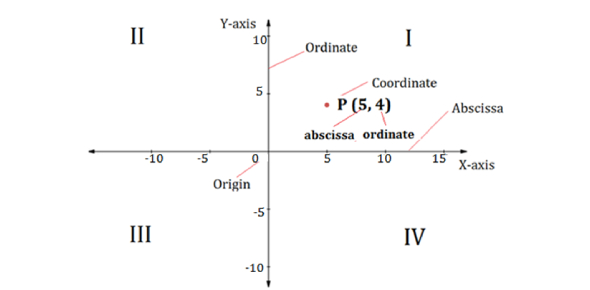# Can You Pass The Coordinate Geometry Quiz?

10 Questions | Attempts: 897
ShareSettingsCoordinate geometry is a branch of mathematics representing the points, lines, and geometric figures in the coordinate system and studying their geometric properties. It helps with solving many problems that come up in common everyday situations. Coordinate geometry is described as the study of geometry using coordinate points. Test your knowledge of coordinate geometry with this fantastic quiz.

• 1.
If (4,1) is the midpoint of the interval from (x,-2) to (5,y), what is the value of y?
• A.

0

• B.

6

• C.

-3

• D.

-10

• E.

4

• 2.
The line through the point (-3,-5) parallel to the x-axis has the equation.
• A.

X=-3

• B.

X=-5

• C.

Y=-3

• D.

Y=-5

• E.

5x=3y

• 3.
Given 3 points, P, Q, and R with R on the x-axis, the equation of RQ is y=2x-1. if QP is parallel to the x-axis and the coordinates of P is (8,4), then the distance from P to Q is:
• A.

3.5

• B.

4

• C.

4.5

• D.

5

• E.

5.5

• 4.
The equation of the straight line passing through (3,5) perpendicular to 3x+y=6 is:
• A.

3y+x=6

• B.

3y-x-12=0

• C.

3y+x=18

• D.

3y+x+6=0

• E.

3y-x-18=0

• 5.
The point (-2,4) is the mid point of the line segment PQ where P is the point (2,-2). The coordinates of Q are:
• A.

(0,1)

• B.

(-6,6)

• C.

(6,-6)

• D.

(-2,6)

• E.

(-6,10)

• 6.
If the line y=2x+3 is reflected in the line y=x+1, its equation becomes
• A.

2x-y=0

• B.

X-2y+1=0

• C.

X-2y+0

• D.

X-2y-2=0

• E.

2x-2y-2+0

• 7.
The x-coordinate of the foot of the perpendicular from the point(1,9) to the line y=x is
• A.

4

• B.

5

• C.

6

• D.

7

• E.

9

• 8.
The points (-1,6), (0,0) and (3,1) are three vertices of a parallelogram. The number of possible positions of the fourth vertex is
• A.

0

• B.

1

• C.

2

• D.

3

• E.

4

• 9.
The gradient of a straight line is -3/2 and it cuts the x-axis at the point (4,0). The equation of the line is
• A.

2y-3x=6

• B.

2y=-3x+12

• C.

2y=3x+8

• D.

2y=3x-12

• E.

2y=3x-8

• 10.
If the point (x,y) is first reflected in the line x=0 and the resulting point is then reflected in the line y=0, then the image point has coordinates
• A.

(x,1-y)

• B.

(0,1)

• C.

(-x,1-y)

• D.

(-x, -y)

• E.

(y,-x)

## Related TopicsBack to top
×

Wait!
Here's an interesting quiz for you.